# Calculated total in charts

Diogo Thomaz shared this problem ago
Resolved

Hello,

I am preparing reports where I use calculated fields to get averages or other values. It works well and I then use the Calculated total in the Totals option, and it works well.

However I then need to prepare a chart that is a Numeric Display showing this calculated total and I don't see this option. I see the total or the average or a count but not the calculated total. When one aggregates data and wants to show a number (KPI) in the form of a Numeric Display the calculated total is the true value we should be showing. How can I do this? Meaning, how can I get a Numeric Display chart showing this calculated total?

The same applies to other chart types such as the Meter, Thermometer or Dial.

We use the March 2018 built of 7.4.

Thank you,

Diogo1

Hi Diogo,

Thanks for reaching out. This is indeed an already logged Defect. There's currently no way of getting to this value and there's no way around this as of now, other than reaching the Calculated Total value in another fashion, perhaps by creating a Calculated Field to reach your Calculated Total value, depending on your data set and use case of course.

I've added you to our client list of those experiencing this behavior in our internal task. I apologize for any inconvenience this may cause. Any potential updates regarding this will be posted here.

Regards,

Mike1

OK Mike, thanks for the reply.

We'll wait although I think this is a priority issue as many users for sure want to report KPIs from these calculated totals.

Best,

Diogo1

Hi Diogo,

Thanks for the feedback. This sounds a little different than simply having the option to use a Calculated Total in the charts section. Can you send a screenshot of where in the application you'd like to see the Calculated Total as an option, as it relates to KPI? I'm not 100% sure on this point.

Thanks,

Mike1

Hello Mike,

In fish farming FCR (kg of feed used to produce 1kg of fish) is the main production performance KPI.

I have a report that summarises for all tanks of all farms of a company the FCR for the week. The data looks like this: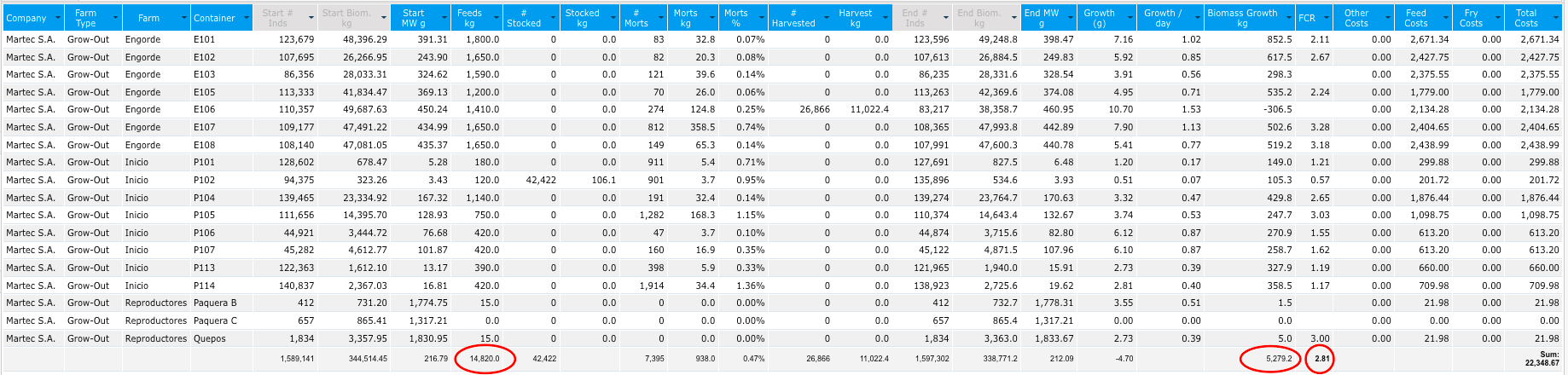The FCR shown is a calculated field: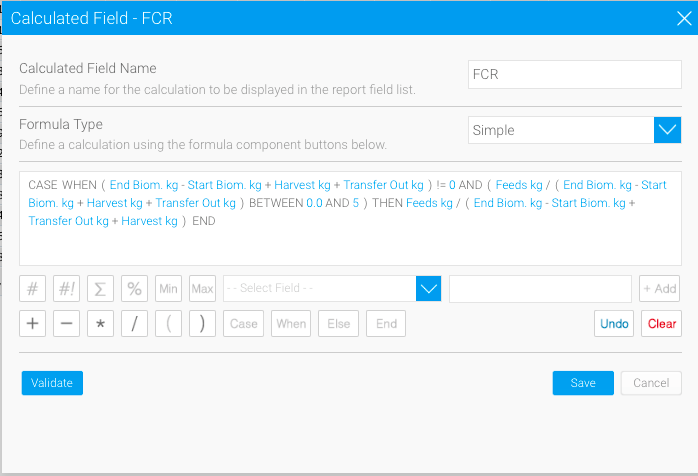and at the bottom I use the Calculated Total as the summary: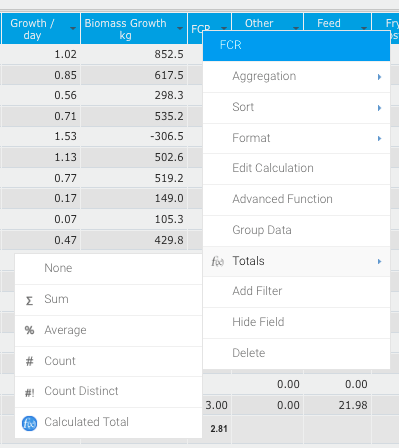The result is 2.81 and it is correct; if you divide the total feeds used (circled above) by the total biomass growth (also circled) you get 2.81.

However, if you use instead the average to summarise the FCR you get the value of 2.11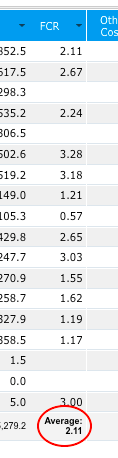As this is a key KPI I wanted to show it in a nice way so I used a dial chart but as you can see, the value shown is the average and not the calculated total: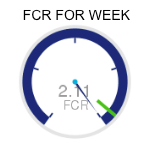In this case the difference is sufficiently large to make the report useless... 2.1 is an excellent result in this particular case and 2.81 is poor to bad.

Not sure if this is clear enough...

Best regards,

Diogo1

Hi Diogo,

Thanks for explaining your use case. This should be helpful in aiding to pursue this functionality. I just wanted to clarify whether you were referencing our KPI feature or not. I see this is indeed related to the Calculated Total's themselves now though.

I've gone ahead and made note that this is high priority for you in our internal task.

Thanks again for the feedback.

Regards,

Mike1

Has this been fixed in any current versions? I'm running into the same issue.

THank you1

Hi Michelle,

Thanks for reaching out. This still occurs. The primary issue here is that calculated totals are not used at the charting level so this is quite an extensive re-write. There have been some recent comments in sub-tasks related to this, so I suspect this to be looked at shortly. In the meantime I've also gone ahead and added you to the client list of those being impacted by this behavior. I'll post any updates in here as they come along.

Regards,

Mike1

Thank you. This affects a lot of KPIs - anytime we want to do an Average Number of Plans per Person, etc - we will be unable to do. Has anybody figured out any work arounds for this?1

Hi Michelle,

You're welcome. There may be a way of getting to your Calculated Total value by other means. There's technically no other way around this as of now other than reaching the Calculated Total value in another fashion, perhaps by creating a Calculated Field. However, the possibility of doing so is dependent on your data set and use case, of course.

Regards,

Mike1

Hi Diogo,

I've realized there actually should be a Calculated Total option for Numeric Display Charts and Dial Charts. You should see the option in Aggregation Type > between Default Aggregate Total and Aggregation: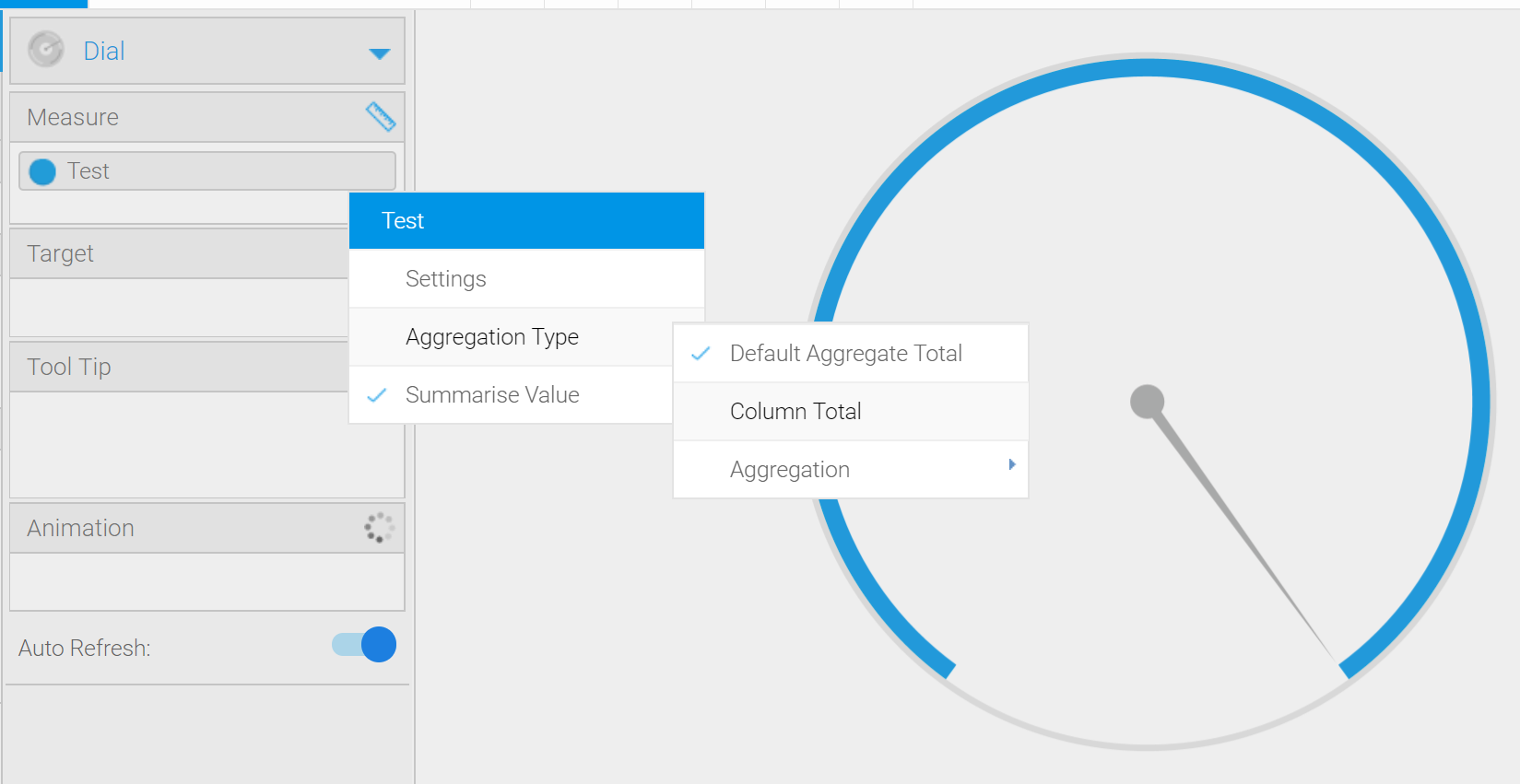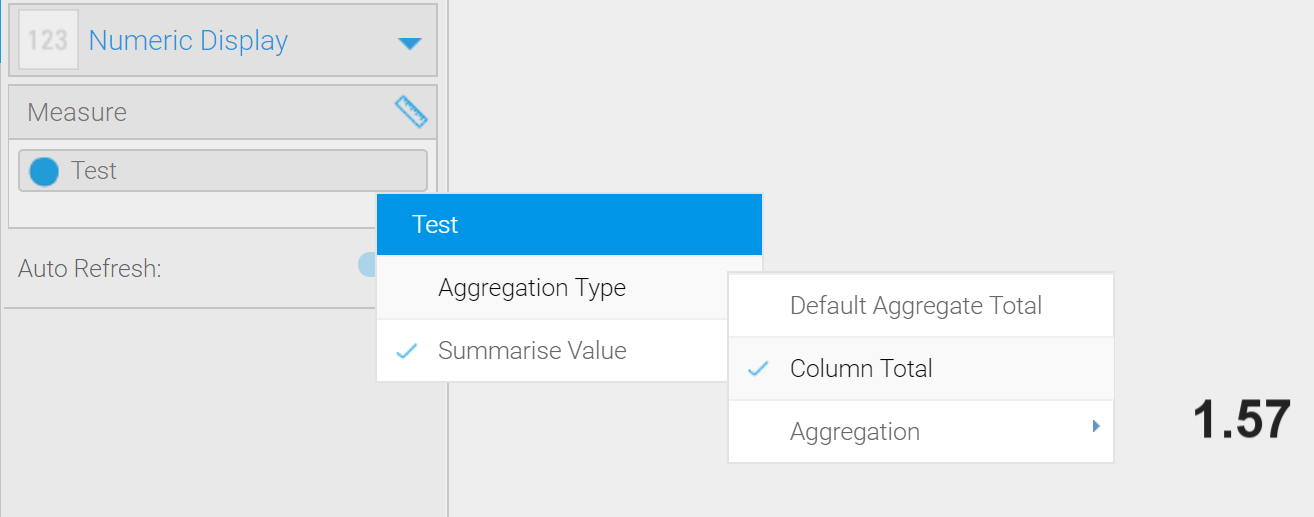The only caveat here is that to have a calculated total, the column total needs to be turned on and has to be a calculated total, on the report. If that isn't turned on, then there will not be the option to use the 'column total'. This is also in 7.4.10, which was published 20190510, so if the option is not available in the March 2018 build of 7.4 and assuming the Calculated Total is being used in the corresponding field at the report level, it should be in latest build of 7.4, as can be seen above.

Regards,

Mike1

Hello Mike,

Thanks for this. It works as expected (I have checked). I don't know why I did not see this before...

I think you can close the issue.

Best regards,

Diogo1

Hi Diogo,

Great! Thanks for confirming.

Please don't hesitate to reach out with any other questions or concerns.Regards,

Mike